Vibrations and Waves - Lesson 0 - Vibrations

# Pendulum Motion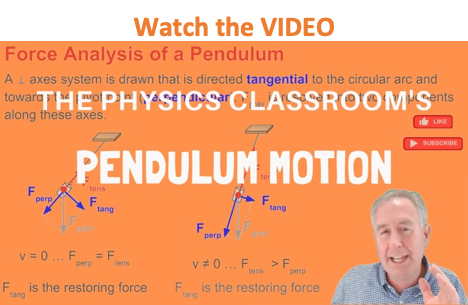A simple pendulum consists of a relatively massive object hung by a string from a fixed support. It typically hangs vertically in its equilibrium position. The massive object is affectionately referred to as the pendulum bob. When the bob is displaced from equilibrium and then released, it begins its back and forth vibration about its fixed equilibrium position. The motion is regular and repeating, an example of periodic motion. Pendulum motion was introduced earlier in this lesson as we made an attempt to understand the nature of vibrating objects. Pendulum motion was discussed again as we looked at the mathematical properties of objects that are in periodic motion. Here we will investigate pendulum motion in even greater detail as we focus upon how a variety of quantities change over the course of time. Such quantities will include forces, position, velocity and energy - both kinetic and potential energy.

### Force Analysis of a Pendulum

Earlier in this lesson we learned that an object that is vibrating is acted upon by a restoring force. The restoring force causes the vibrating object to slow down as it moves away from the equilibrium position and to speed up as it approaches the equilibrium position. It is this restoring force that is responsible for the vibration. So what forces act upon a pendulum bob? And what is the restoring force for a pendulum? There are two dominant forces acting upon a pendulum bob at all times during the course of its motion. There is the force of gravity that acts downward upon the bob. It results from the Earth's mass attracting the mass of the bob. And there is a tension force acting upward and towards the pivot point of the pendulum. The tension force results from the string pulling upon the bob of the pendulum. In our discussion, we will ignore the influence of air resistance - a third force that always opposes the motion of the bob as it swings to and fro. The air resistance force is relatively weak compared to the two dominant forces.

The gravity force is highly predictable; it is always in the same direction (down) and always of the same magnitude - mass*9.8 N/kg. The tension force is considerably less predictable. Both its direction and its magnitude change as the bob swings to and fro. The direction of the tension force is always towards the pivot point. So as the bob swings to the left of its equilibrium position, the tension force is at an angle - directed upwards and to the right. And as the bob swings to the right of its equilibrium position, the tension is directed upwards and to the left. The diagram below depicts the direction of these two forces at five different positions over the course of the pendulum's path.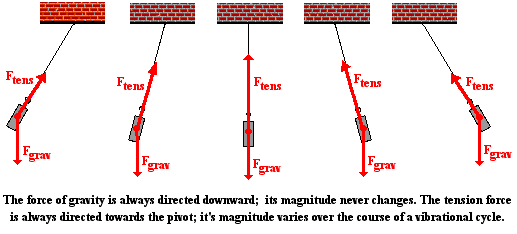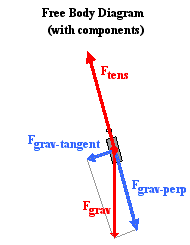In physical situations in which the forces acting on an object are not in the same, opposite or perpendicular directions, it is customary to resolve one or more of the forces into components. This was the practice used in the analysis of sign hanging problems and inclined plane problems. Typically one or more of the forces are resolved into perpendicular components that lie along coordinate axes that are directed in the direction of the acceleration or perpendicular to it. So in the case of a pendulum, it is the gravity force which gets resolved since the tension force is already directed perpendicular to the motion. The diagram at the right shows the pendulum bob at a position to the right of its equilibrium position and midway to the point of maximum displacement. A coordinate axis system is sketched on the diagram and the force of gravity is resolved into two components that lie along these axes. One of the components is directed tangent to the circular arc along which the pendulum bob moves; this component is labeled Fgrav-tangent. The other component is directed perpendicular to the arc; it is labeled Fgrav-perp. You will notice that the perpendicular component of gravity is in the opposite direction of the tension force. You might also notice that the tension force is slightly larger than this component of gravity. The fact that the tension force (Ftens) is greater than the perpendicular component of gravity (Fgrav-perp) means there will be a net force which is perpendicular to the arc of the bob's motion. This must be the case since we expect that objects that move along circular paths will experience an inward or centripetal force. The tangential component of gravity (Fgrav-tangent) is unbalanced by any other force. So there is a net force directed along the other coordinate axes. It is this tangential component of gravity which acts as the restoring force. As the pendulum bob moves to the right of the equilibrium position, this force component is directed opposite its motion back towards the equilibrium position.

The above analysis applies for a single location along the pendulum's arc. At the other locations along the arc, the strength of the tension force will vary. Yet the process of resolving gravity into two components along axes that are perpendicular and tangent to the arc remains the same. The diagram below shows the results of the force analysis for several other positions.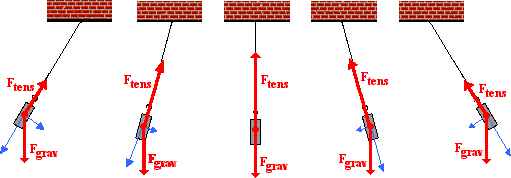There are a couple comments to be made. First, observe the diagram for when the bob is displaced to its maximum displacement to the right of the equilibrium position. This is the position in which the pendulum bob momentarily has a velocity of 0 m/s and is changing its direction. The tension force (Ftens) and the perpendicular component of gravity (Fgrav-perp) balance each other. At this instant in time, there is no net force directed along the axis that is perpendicular to the motion. Since the motion of the object is momentarily paused, there is no need for a centripetal force.

Second, observe the diagram for when the bob is at the equilibrium position (the string is completely vertical). When at this position, there is no component of force along the tangent direction. When moving through the equilibrium position, the restoring force is momentarily absent. Having been restored to the equilibrium position, there is no restoring force. The restoring force is only needed when the pendulum bob has been displaced away from the equilibrium position. You might also notice that the tension force (Ftens) is greater than the perpendicular component of gravity (Fgrav-perp) when the bob moves through this equilibrium position. Since the bob is in motion along a circular arc, there must be a net centripetal force at this position.

### The Sinusoidal Nature of Pendulum Motion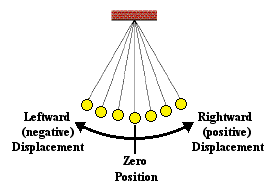In the previous part of this lesson, we investigated the sinusoidal nature of the motion of a mass on a spring. We will conduct a similar investigation here for the motion of a pendulum bob. Let's suppose that we could measure the amount that the pendulum bob is displaced to the left or to the right of its equilibrium or rest position over the course of time. A displacement to the right of the equilibrium position would be regarded as a positive displacement; and a displacement to the left would be regarded as a negative displacement. Using this reference frame, the equilibrium position would be regarded as the zero position. And suppose that we constructed a plot showing the variation in position with respect to time. The resulting position vs. time plot is shown below. Similar to what was observed for the mass on a spring, the position of the pendulum bob (measured along the arc relative to its rest position) is a function of the sine of the time.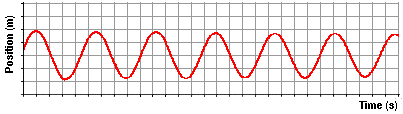Now suppose that we use our motion detector to investigate the how the velocity of the pendulum changes with respect to the time. As the pendulum bob does the back and forth, the velocity is continuously changing. There will be times at which the velocity is a negative value (for moving leftward) and other times at which it will be a positive value (for moving rightward). And of course there will be moments in time at which the velocity is 0 m/s. If the variations in velocity over the course of time were plotted, the resulting graph would resemble the one shown below.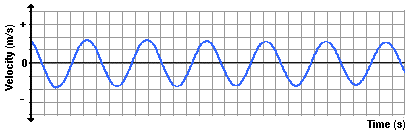Now let's try to understand the relationship between the position of the bob along the arc of its motion and the velocity with which it moves. Suppose we identify several locations along the arc and then relate these positions to the velocity of the pendulum bob. The graphic below shows an effort to make such a connection between position and velocity.As is often said, a picture is worth a thousand words. Now here come the words. The plot above is based upon the equilibrium position (D) being designated as the zero position. A displacement to the left of the equilibrium position is regarded as a negative position. A displacement to the right is regarded as a positive position. An analysis of the plots shows that the velocity is least when the displacement is greatest. And the velocity is greatest when the displacement of the bob is least. The further the bob has moved away from the equilibrium position, the slower it moves; and the closer the bob is to the equilibrium position, the faster it moves. This can be explained by the fact that as the bob moves away from the equilibrium position, there is a restoring force that opposes its motion. This force slows the bob down. So as the bob moves leftward from position D to E to F to G, the force and acceleration is directed rightward and the velocity decreases as it moves along the arc from D to G. At G - the maximum displacement to the left - the pendulum bob has a velocity of 0 m/s. You might think of the bob as being momentarily paused and ready to change its direction. Next the bob moves rightward along the arc from G to F to E to D. As it does, the restoring force is directed to the right in the same direction as the bob is moving. This force will accelerate the bob, giving it a maximum speed at position D - the equilibrium position. As the bob moves past position D, it is moving rightward alo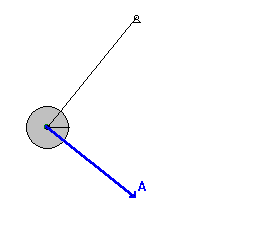ng the arc towards C, then B and then A. As it does, there is a leftward restoring force opposing its motion and causing it to slow down. So as the displacement increases from D to A, the speed decreases due to the opposing force. Once the bob reaches position A - the maximum displacement to the right - it has attained a velocity of 0 m/s. Once again, the bob's velocity is least when the displacement is greatest. The bob completes its cycle, moving leftward from A to B to C to D. Along this arc from A to D, the restoring force is in the direction of the motion, thus speeding the bob up. So it would be logical to conclude that as the position decreases (along the arc from A to D), the velocity increases. Once at position D, the bob will have a zero displacement and a maximum velocity. The velocity is greatest when the displacement is least. The animation at the right (used with the permission of Wikimedia Commons; special thanks to Hubert Christiaen) provides a visual depiction of these principles. The acceleration vector that is shown combines both the perpendicular and the tangential accelerations into a single vector. You will notice that this vector is entirely tangent to the arc when at maximum displacement; this is consistent with the force analysis discussed above. And the vector is vertical (towards the center of the arc) when at the equilibrium position. This also is consistent with the force analysis discussed above.

### Energy Analysis

In a previous chapter of The Physics Classroom Tutorial, the energy possessed by a pendulum bob was discussed. We will expand on that discussion here as we make an effort to associate the motion characteristics described above with the concepts of kinetic energy, potential energy and total mechanical energy.

The kinetic energy possessed by an object is the energy it possesses due to its motion. It is a quantity that depends upon both mass and speed. The equation that relates kinetic energy (KE) to mass (m) and speed (v) is

KE = ½•m•v2

The faster an object moves, the more kinetic energy that it will possess. We can combine this concept with the discussion above about how speed changes during the course of motion. This blending of concepts would lead us to conclude that the kinetic energy of the pendulum bob increases as the bob approaches the equilibrium position. And the kinetic energy decreases as the bob moves further away from the equilibrium position.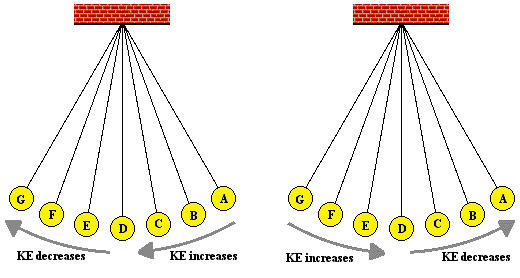The potential energy possessed by an object is the stored energy of position. Two types of potential energy are discussed in The Physics Classroom Tutorial - gravitational potential energy and elastic potential energy. Elastic potential energy is only present when a spring (or other elastic medium) is compressed or stretched. A simple pendulum does not consist of a spring. The form of potential energy possessed by a pendulum bob is gravitational potential energy. The amount of gravitational potential energy is dependent upon the mass (m) of the object and the height (h) of the object. The equation for gravitational potential energy (PE) is

PE = m•g•h

where g represents the gravitational field strength (sometimes referred to as the acceleration caused by gravity) and has the value of 9.8 N/kg.

The height of an object is expressed relative to some arbitrarily assigned zero level. In other words, the height must be measured as a vertical distance above some reference position. For a pendulum bob, it is customary to call the lowest position the reference position or the zero level. So when the bob is at the equilibrium position (the lowest position), its height is zero and its potential energy is 0 J. As the pendulum bob does the back and forth, there are times during which the bob is moving away from the equilibrium position. As it does, its height is increasing as it moves further and further away. It reaches a maximum height as it reaches the position of maximum displacement from the equilibrium position. As the bob moves towards its equilibrium position, it decreases its height and decreases its potential energy.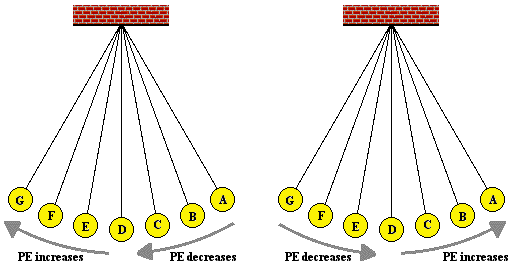Now let's put these two concepts of kinetic energy and potential energy together as we consider the motion of a pendulum bob moving along the arc shown in the diagram at the right. We will use an energy bar chart to represent the changes in the two forms of energy. The amount of each form of energy is represented by a bar. The height of the bar is proportional to the amount of that form of energy. In addition to the potential energy (PE) bar and kinetic energy (KE) bar, there is a third bar labeled TME. The TME bar represents the total amount of mechanical energy possessed by the pendulum bob. The total mechanical energy is simply the sum of the two forms of energy – kinetic plus potential energy. Take some time to inspect the bar charts shown below for positions A, B, D, F and G. What do you notice?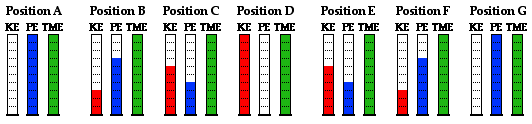When you inspect the bar charts, it is evident that as the bob moves from A to D, the kinetic energy is increasing and the potential energy is decreasing. However, the total amount of these two forms of energy is remaining constant. Whatever potential energy is lost in going from position A to position D appears as kinetic energy. There is a transformation of potential energy into kinetic energy as the bob moves from position A to position D. Yet the total mechanical energy remains constant. We would say that mechanical energy is conserved. As the bob moves past position D towards position G, the opposite is observed. Kinetic energy decreases as the bob moves rightward and (more importantly) upward toward position G. There is an increase in potential energy to accompany this decrease in kinetic energy. Energy is being transformed from kinetic form into potential form. Yet, as illustrated by the TME bar, the total amount of mechanical energy is conserved. This very principle of energy conservation was explained in the Energy chapter of The Physics Classroom Tutorial.

### The Period of a Pendulum

Our final discussion will pertain to the period of the pendulum. As discussed previously in this lesson, the period is the time it takes for a vibrating object to complete its cycle. In the case of pendulum, it is the time for the pendulum to start at one extreme, travel to the opposite extreme, and then return to the original location. Here we will be interested in the question What variables affect the period of a pendulum? We will concern ourselves with possible variables. The variables are the mass of the pendulum bob, the length of the string on which it hangs, and the angular displacement. The angular displacement or arc angle is the angle that the string makes with the vertical when released from rest. These three variables and their effect on the period are easily studied and are often the focus of a physics lab in an introductory physics class. The data table below provides representative data for such a study.

 Trial Mass (kg) Length (m) Arc Angle (°) Period (s) 1 0.02- 0.40 15.0 1.25 2 0.050 0.40 15.0 1.29 3 0.100 0.40 15.0 1.28 4 0.200 0.40 15.0 1.24 5 0.500 0.40 15.0 1.26 6 0.200 0.60 15.0 1.56 7 0.200 0.80 15.0 1.79 8 0.200 1.00 15.0 2.01 9 0.200 1.20 15.0 2.19 10 0.200 0.40 10.0 1.27 11 0.200 0.40 20.0 1.29 12 0.200 0.40 25.0 1.25 13 0.200 0.40 30.0 1.26

In trials 1 through 5, the mass of the bob was systematically altered while keeping the other quantities constant. By so doing, the experimenters were able to investigate the possible effect of the mass upon the period. As can be seen in these five trials, alterations in mass have little effect upon the period of the pendulum.

In trials 4 and 6-9, the mass is held constant at 0.200 kg and the arc angle is held constant at 15°. However, the length of the pendulum is varied. By so doing, the experimenters were able to investigate the possible effect of the length of the string upon the period. As can be seen in these five trials, alterations in length definitely have an effect upon the period of the pendulum. As the string is lengthened, the period of the pendulum is increased. There is a direct relationship between the period and the length.

Finally, the experimenters investigated the possible effect of the arc angle upon the period in trials 4 and 10-13. The mass is held constant at 0.200 kg and the string length is held constant at 0.400 m. As can be seen from these five trials, alterations in the arc angle have little to no effect upon the period of the pendulum.

So the conclusion from such an experiment is that the one variable that effects the period of the pendulum is the length of the string. Increases in the length lead to increases in the period. But the investigation doesn't have to stop there. The quantitative equation relating these variables can be determined if the data is plotted and linear regression analysis is performed. The two plots below represent such an analysis. In each plot, values of period (the dependent variable) are placed on the vertical axis. In the plot on the left, the length of the pendulum is placed on the horizontal axis. The shape of the curve indicates some sort of power relationship between period and length. In the plot on the right, the square root of the length of the pendulum (length to the ½ power) is plotted. The results of the regression analysis are shown.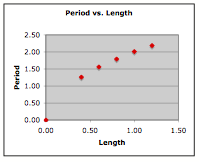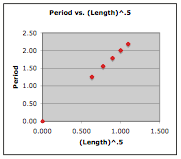Slope: 1.7536 Y-intercept: 0.2616 COR: 0.9183 Slope: 2.0045 Y-intercept: 0.0077 COR: 0.9999

The analysis shows that there is a better fit of the data and the regression line for the graph on the right. As such, the plot on the right is the basis for the equation relating the period and the length. For this data, the equation is

Period = 2.0045•Length0.5 + 0.0077

Using T as the symbol for period and L as the symbol for length, the equation can be rewritten as

T = 2.0045•L0.5 + 0.0077

The commonly reported equation based on theoretical development is

T = 2•Π•(L/g)0.5

where g is a constant known as the gravitational field strength or the acceleration of gravity (9.8 N/kg). The value of 2.0045 from the experimental investigation agrees well with what would be expected from this theoretically reported equation. Substituting the value of g into this equation, yields a proportionality constant of 2Π/g0.5, which is 2.0071, very similar to the 2.0045 proportionality constant developed in the experiment.

### Investigate!

Use the Investigating a Pendulum widget below to investigate the effect of the pendulum length upon the period of the pendulum. Simply type in a value of length into the input field and click on the Submit button. Experiment with various values of pendulum length.

### We Would Like to Suggest ...Why just read about it and when you could be interacting with it? Interact - that's exactly what you do when you use one of The Physics Classroom's Interactives. We would like to suggest that you combine the reading of this page with the use of our Pendulum Motion Interactive. You can find it in the Physics Interactives section of our website.

Visit: Pendulum Motion Interactive

1. A pendulum bob is pulled back to position A and released from rest. The bob swings through its usual circular arc and is caught at position C. Determine the position (A, B, C or all the same) where the …

a. … force of gravity is the greatest?
b. … restoring force is the greatest?
c. … speed is the greatest?
d. … potential energy is the greatest?
e. … kinetic energy is the greatest
f. … total mechanical energy is the greatest?

2. Use energy conservation to fill in the blanks in the following diagram.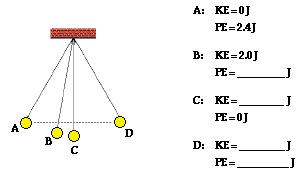3. A pair of trapeze performers at the circus is swinging from ropes attached to a large elevated platform. Suppose that the performers can be treated as a simple pendulum with a length of 16 m. Determine the period for one complete back and forth cycle.

4. Which would have the highest frequency of vibration?

Pendulum A: A 200-g mass attached to a 1.0-m length string
Pendulum B: A 400-g mass attached to a 0.5-m length string

5. Anna Litical wishes to make a simple pendulum that serves as a timing device. She plans to make it such that its period is 1.00 second. What length must the pendulum have?

Next Section: